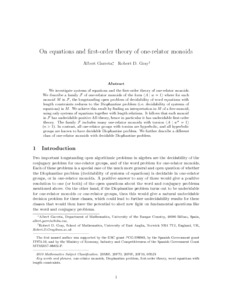# On equations and first-order theory of one-relator monoids

Garreta, Albert and Gray, Robert (2021) On equations and first-order theory of one-relator monoids. Information and Computation, 281. ISSN 0890-5401Preview PDF (GarretaGrayIandCPaperFinal5April2021) - Accepted Version Available under License Creative Commons Attribution Non-commercial No Derivatives. Download (523kB) | Preview

## Abstract

We investigate systems of equations and the first-order theory of one-relator monoids. We describe a family F of one-relator monoids of the form 〈A|w=1〉 where for each monoid M in F, the longstanding open problem of decidability of word equations with length constraints reduces to the Diophantine problem (i.e. decidability of systems of equations) in M. We achieve this result by finding an interpretation in M of a free monoid, using only systems of equations together with length relations. It follows that each monoid in F has undecidable positive AE-theory, hence in particular it has undecidable first-order theory. The family F includes many one-relator monoids with torsion 〈A|w n=1〉 (n>1). In contrast, all one-relator groups with torsion are hyperbolic, and all hyperbolic groups are known to have decidable Diophantine problem. We further describe a different class of one-relator monoids with decidable Diophantine problem.

Item Type: Article diophantine problem,first-order theory,one-relator monoids,word equations with length constraints,theoretical computer science,information systems,computer science applications,computational theory and mathematics ,/dk/atira/pure/subjectarea/asjc/2600/2614 Faculty of Science > School of Mathematics Faculty of Science > Research Groups > Algebra and CombinatoricsFaculty of Science > Research Groups > Logic http://www.scopus.com/inward/record.url?... LivePure Connector 15 Apr 2021 23:51 14 May 2023 00:53 https://ueaeprints.uea.ac.uk/id/eprint/79796 10.1016/j.ic.2021.104745View Item